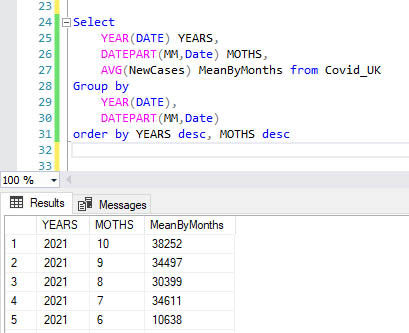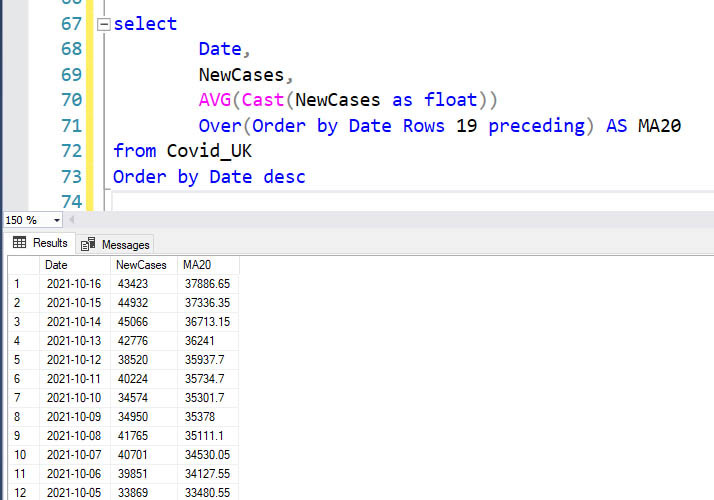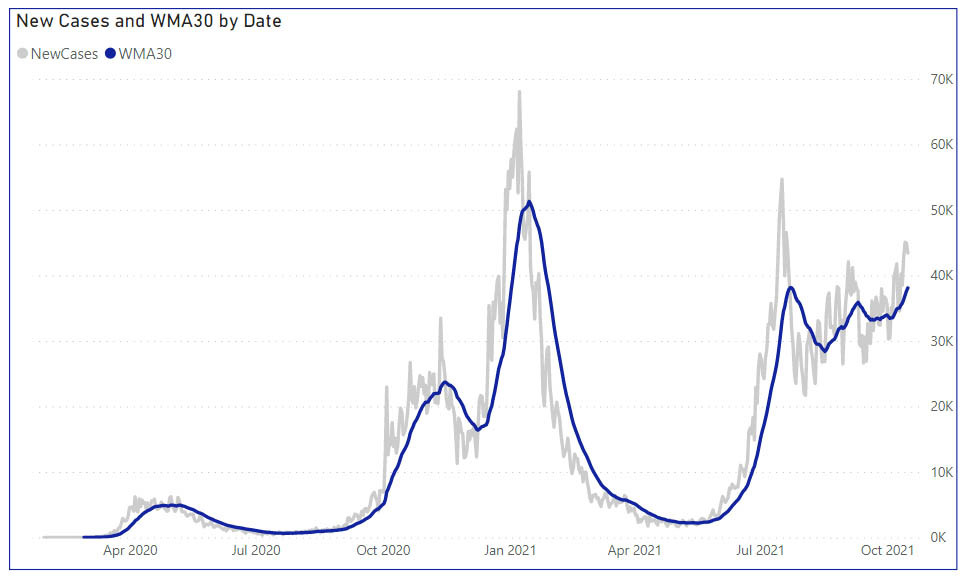Search
• Marek Vavrovic

# Calculating Average using T-SQL

For the first couple of exercises, I will be using the covid-19 data set from the government website. My first task is to calculate the mean by week using t-sql language.Example 1

## Calculating average by week number.

For this calculation I am using DATEPART() function to return the week numbers. This function returns an integer that represents the specified datepart of the specified date. There is one more similar function to this one, DATENAME() which returns a string and string can not be sorted.

Select

YEAR(DATE) YEARS,

DATEPART(WW,Date) WEEKS,

AVG(NewCases) MeanByWeek from Covid_UK

Group by

YEAR(DATE),

DATEPART(WW,Date)

order by YEARS desc, WEEKS descExample 3

## Calculating average by month number.

A similar calculation as in the previous case, the only thing I needed to change is the interval in DATEPART(MM, Date) function.Example 4

## Using AVG() OVER()

Let's say I want to see both calculations as a part of one result. I want to create a table that includes new cases by date, average by week number, and average by month number. To achieve this, I will be using AVG() OVER() function, which is a part of the window functions.

When you specify a column or set of columns with the PARTITION BY clause, then it will divide the result set into record partitions. Then, finally AVG() function is applied to each record partition separately.

Select

date,

YEAR(DATE) YEARS,

DATEPART(MM,Date) MONTHS,

DATEPART(WW,Date) WEEKS,

NewCases,

AVG(NewCases) OVER(PARTITION BY YEAR(DATE) , DATEPART(WW,Date)

Order by YEAR(DATE),DATEPART(WW,Date) ) as MeanByWeek,

AVG(NewCases) OVER(PARTITION BY YEAR(DATE) , DATEPART(MM,Date)

Order by YEAR(DATE),DATEPART(MM,Date) ) as MeanByMonth

from Covid_UK

order by date descIf you plot this, you can see that the new cases are slightly increasing in the last two months.Example 5

## Moving average in T-SQL

There are two moving averages used with technical analysis in the Financial Markets - Simple and Exponential. The goal here is to provide you with a solution that will allow you to do Simple Moving Averages (SMA) efficiently. The moving average shows a smoother curve than the actual values, and it is a good tool for trend analysis.

Select

Date,

NewCases,

AVG(NewCases)

Over(Order by Date Rows 19 Preceding) AS MA20

From Covid_UK

Rows 19 preceding means start at the current row and include next 19 rows. Hence 1 current row + 19 preceding rows =20The figures are displayed as integer. If you want to use decimals, you have to cast NewCases values.The code bellow is displaying new cases, 20-day moving average and 60-day moving average.

Moving averages are applied as a tool to demonstrate trends. A popular approach is to combine moving averages of different intervals, to spot variations in the short-, medium- and long-term trends respectively. Of particular interest are the crossing of trend lines. For instance, when the short trend moves over the long or medium trend, this can be interpreted as a buy signal in technical analysis. And when the short trend moves under a longer trend line, this can be interpreted as a sell signal.

Select

Date,

NewCases,

AVG(NewCases)

Over(Order by Date Rows 19 preceding) AS MA20,

AVG(NewCases)

Over(Order by Date Rows 59 preceding) AS MA60

From Covid_UKExample 6

## Weighted Moving Average

sample stock dataWeighted moving average calculation = (Price * weighting factor) + (Price previous period * weighting factor-1) WMAs can have different weights assigned based on the number of periods used in the calculation.

In the example I will calculate weighted moving average for 9 days (WMA9). The weight for each row will be calculated by using the window row position relative to the current row. Let’s say I want to calculate WMA for the 9th row.

1. multiply Index * close_price

2. The sum of the above is The sum of the above is 24381,95. This is then divided by the sum of the weights, that is 1+2+3+4+5+6+7+8+9 = 45. The WMA9 for row 9 is 24381,95 / 45 = 541,8211WITH Prices AS

(

SELECT

quote_date,

ROW_NUMBER() Over(Order by quote_date) as QouteIndex

,close_price

FROM MavaStock

)

SELECT

P.quote_date

,P.QouteIndex

,P.close_price

,CASE WHEN P.QouteIndex >= 9 THEN SUM((WMA9.QouteIndex - p.QouteIndex + 9.0) * WMA9.close_price) / 45.0 END AS WMA9

FROM

Prices AS P

LEFT OUTER JOIN

Prices AS WMA9

on

WMA9.QouteIndex <= p.QouteIndex AND WMA9.QouteIndex >= p.QouteIndex - 9

GROUP BY p.QouteIndex , P.quote_date , P.close_priceCalculating 30-day weighted moving average for covid-19 new cases in the UK.

In case you have more countries in the table use this syntax after specifying LEFT JOIN

ON uk.Country = cuk.Country AND cuk._Index <= uk._Index AND cuk._Index >= uk._Index - 30

With CovidUk

as

(

Select ROW_NUMBER() over(order by date) as _Index,

AreaName, Date, NewCases from Covid_UK

)

Select

uk._Index,

uk.AreaName,

uk.Date,

uk.NewCases,

CASE WHEN uk._Index >= 30 THEN SUM((cuk._Index - uk._Index + 30) * cuk.NewCases) / 465 END AS WMA30

from CovidUk uk

LEFT JOIN CovidUk cuk

ON cuk._Index <= uk._Index AND cuk._Index >= uk._Index - 30

GROUP BY uk._Index,uk.AreaName,uk.Date,uk.NewCasesExample 6

## Smoothed Moving Average

To clarify the long term trend, a technique called smoothing can be used where groups of values are averaged. The graph of moving mean or moving medians is "flatter" than the time series graph with its peaks and troughs. The average can be either a moving mean or a moving median. In this process the mean or median of groups of values are taken.

This simple example created in MS Excel demonstrates how the 3-mean smoothing works.How to achieve this with t-sql?

By using a window function AVG() OVER()

with: ROWS BETWEEN 1 PRECEDING AND 1 FOLLOWING

Calculating 3-Mean Smoothed using t-sql

Select SalesYear,

Sales,

COUNT(Sales) over(order by salesYear ROWS BETWEEN 1 PRECEDING AND 1 FOLLOWING) AS [GroupsBy3],

AVG(Sales)over(order by salesYear ROWS BETWEEN 1 PRECEDING AND 1 FOLLOWING) AS [3-Mean Smoothed]

from SmoothingDataCalculating 5-Mean Smoothed using t-sql

With Sales

AS

(

Select

ROW_NUMBER() OVER(ORDER BY SalesYear) as _Index,

SalesYear,

Sales,

AVG(Cast(Sales as float))over(order by SalesYear

ROWS BETWEEN 2 PRECEDING AND 2 FOLLOWING) AS [5-Mean Smoothed]

From SmoothingData

)

Select

SalesYear, Sales,

CASE WHEN

_Index IN (Select Top 2 _Index from Sales Order by _Index DESC)

OR

_Index IN (Select Top 2 _Index from Sales Order by _Index ASC)

THEN NULL

ELSE [5-Mean Smoothed]

END [5-Mean Smoothed]

From SalesThis is how the data appears on the visual.Calculating 5-Mean Smoothed using variables.

-variables must be declared outside the CTE

-SELECT TOP N is considered as a " performance killer" and variables should improve it. But this is already running in the memory, thanks to ROWS BETWEEN 2 PRECEDING AND 2 FOLLOWING window framing so in this particular case using variables won't improve the performance. In other cases they would do so.

Declare @Nr INT =2

;With Sales

AS

(

Select

ROW_NUMBER() OVER(ORDER BY SalesYear) as _Index,

SalesYear,

Sales,

AVG(Cast(Sales as float))over(order by salesYear

ROWS BETWEEN 2 PRECEDING AND 2 FOLLOWING) AS [5-Mean Smoothed]

From SmoothingData

)

Select

SalesYear, Sales,

CASE WHEN

_Index IN (Select Top (@Nr) _Index from Sales Order by _Index DESC)

OR

_Index IN (Select Top (@Nr) _Index from Sales Order by _Index ASC)

THEN NULL ELSE [5-Mean Smoothed] END [5-Mean Smoothed]

From Sales

Both queries are performing the same way.I will combine smoothing averages of different intervals, to spot variations in the trends respectively for the covid-19 UK data by calculating 7 and 21 mean smoothed.

WITH UKCovidData

AS

(

Select

Date, NewCases,

ROW_NUMBER() OVER(ORDER BY Date) as RN,

AVG(NewCases) OVER(ORDER BY Date

ROWS BETWEEN 3 PRECEDING AND 3 FOLLOWING) AS [7-MeanSmoothed],

AVG(NewCases) OVER(ORDER BY Date

ROWS BETWEEN 10 PRECEDING AND 10 FOLLOWING) AS [21-MeanSmoothed]

From Covid_UK

)

Select Date, NewCases,

CASE WHEN

RN IN (Select Top 3 RN from UKCovidData Order by RN DESC)

OR

RN IN (Select Top 3 RN from UKCovidData Order by RN ASC)

THEN NULL ELSE [7-MeanSmoothed] END [7-Mean Smoothed],

CASE WHEN

RN IN (Select Top 10 RN from UKCovidData Order by RN DESC)

OR

RN IN (Select Top 10 RN from UKCovidData Order by RN ASC)

THEN NULL ELSE [21-MeanSmoothed] END [21-Mean Smoothed]

From UKCovidData

Order by Date descAs you can see from this visual, new cases are slightly increasing. And because there is a strong positive correlation between new cases and hospital cases or between new cases and deaths, the hospital cases and deaths will be slightly increasing as well. If you compare the same period with the previous year, you will find out that there is a double difference in the number of new cases. The most difficult period will come in early January.